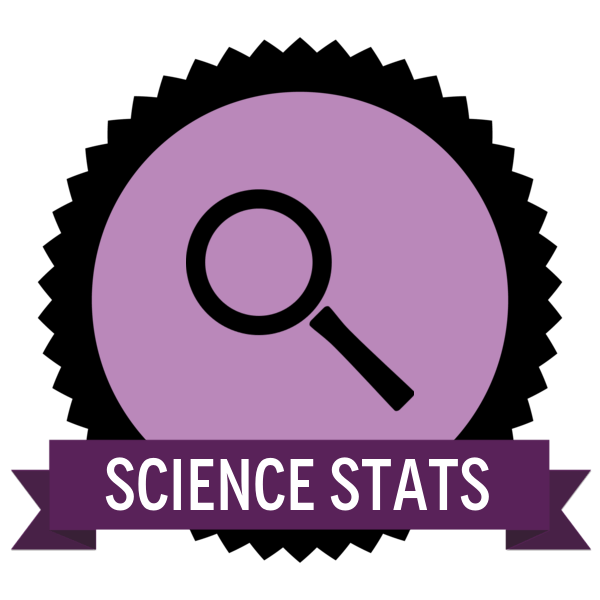# Statistics for Science

0 Points

Why use Statistics in Science?

It’s easy to compartmentalise the subjects we study at school but Maths and Science are two that you just can’t separate. To make our evidence valid we must collect a lot of data and where there’s numerical data, there must come a little maths. When you have a lot of data things can get very complex and it’s easy to lose data quality. Statistical techniques can help us to make the volume of data usable and also allow us to test its validity and reliability.

What is required?

To collect the Statistics for Science Badge you need to:

• Calculate mean values of some data
• Calculate the range of this data
• Display the means and ranges graphically using crosses and range bars
• Explain the significance of the range bars in relation to the means

Make sure the means are recorded to the same number of decimal places as the raw data in your results table.

Follow